# Understanding Linear Equations Chapter Exam

Exam Instructions:

Choose your answers to the questions and click 'Next' to see the next set of questions. You can skip questions if you would like and come back to them later with the yellow "Go To First Skipped Question" button. When you have completed the practice exam, a green submit button will appear. Click it to see your results. Good luck!

### Page 1

#### Question 1 1. If the graph below has the equation Ax + By = -4, what must be true about A and B?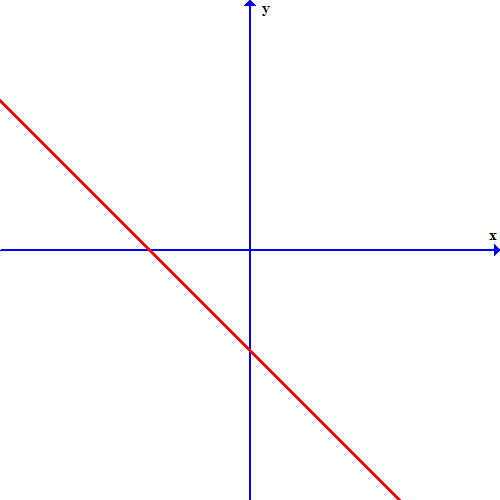### Page 2

#### Question 10 10. Which of the following is a solution to the system of equations shown in the graph below?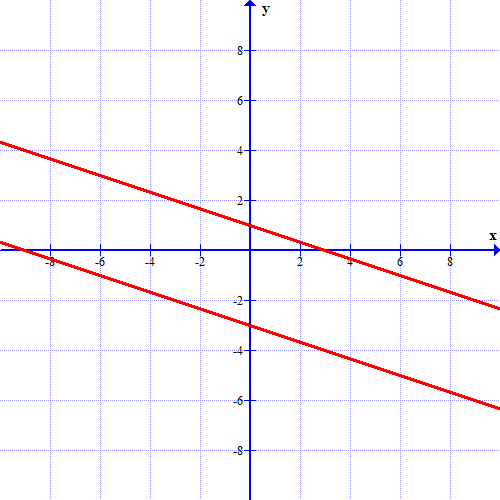### Page 3

#### Question 11 11. Which one of the following equations could work for the following graph?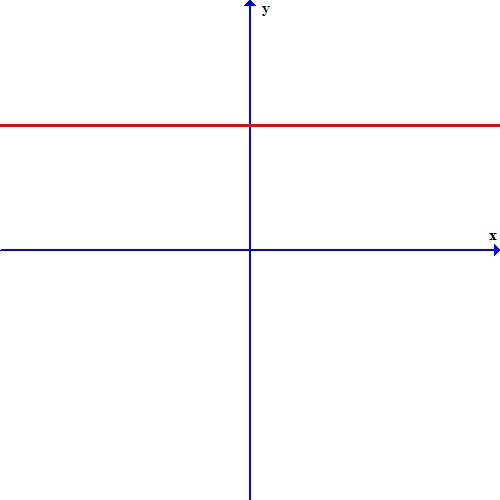#### Question 14 14. What is the equation of the graph below?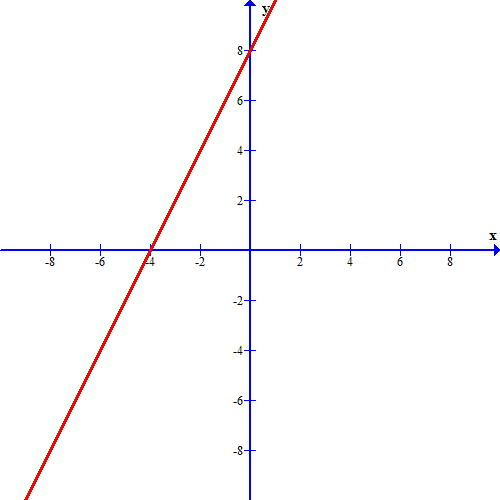### Page 5

#### Question 23 23. Which of these equations represents a line that is perpendicular to the line in the graph below?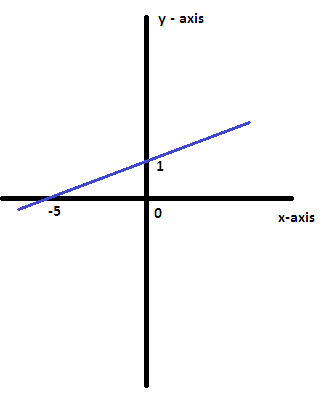#### Question 24 24. If the graph below has the equation Ax+By=C, which statement MUST be true?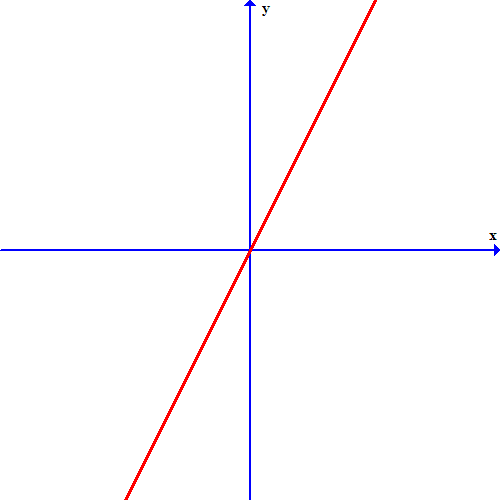### Page 6

#### Question 28 28. If the graph below was written in the slope-intercept form, which of the following would be true?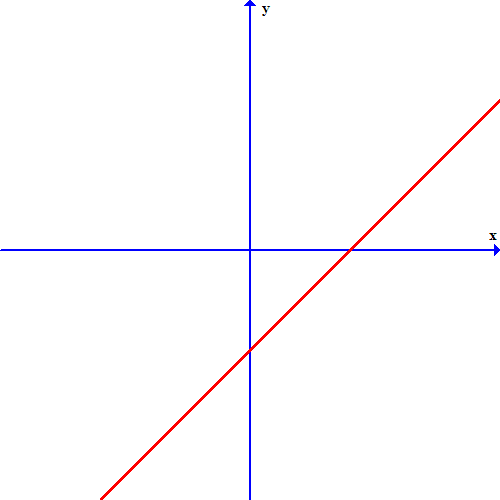#### Question 30 30. Which line is the transversal?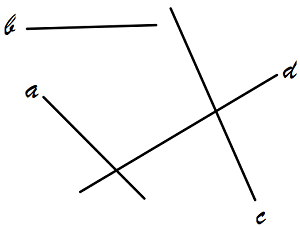#### Understanding Linear Equations Chapter Exam Instructions

Choose your answers to the questions and click 'Next' to see the next set of questions. You can skip questions if you would like and come back to them later with the yellow "Go To First Skipped Question" button. When you have completed the practice exam, a green submit button will appear. Click it to see your results. Good luck!

Support